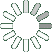Puzzle Command
Daily Puzzle
Number Logic #6
December 14, 2014Find a 6 digit number where:

The 1st digit is equal to 7.
The 2nd digit is the sum of the 1st, 3rd, and 4th digit.
The 3rd digit is an odd number.
The 4th digit is an odd number.
The 5th digit is equal to 9.
The 6th digit is the number of 1s in the number.

Every digit is an odd number.

Puzzle Notes
6 digit numbers range from 100,000 to 999,999.
Every digit is an odd number.
Enter in your solution below and click submit.